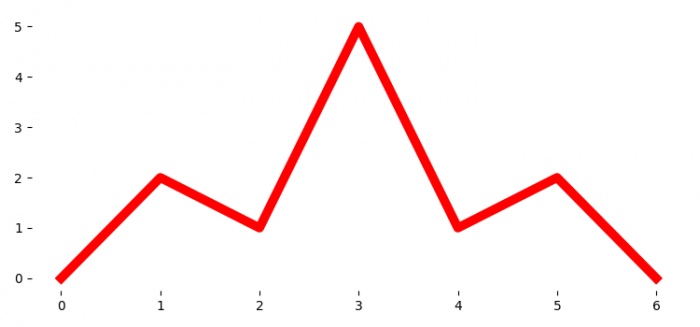# How to remove a frame without removing the axes tick labels from a Matplotlib figure in Python?

To remove a frame without removing the axes tick labels from a Matplotlib figure, we can take the following steps −

• Set the figure size and adjust the padding between and around the subplots.
• Create a list of y data points.
• Plot the y data points using plot() method
• To remove the left-right-top and bottom spines, we can use set_visible() method.
• To display the figure, use show() method.

## Example

import matplotlib.pyplot as plt

plt.rcParams["figure.figsize"] = [7.50, 3.50]
plt.rcParams["figure.autolayout"] = True

y = [0, 2, 1, 5, 1, 2, 0]

plt.plot(y, color='red', lw=7)

for pos in ['right', 'top', 'bottom', 'left']:
plt.gca().spines[pos].set_visible(False)

plt.show()

## Output